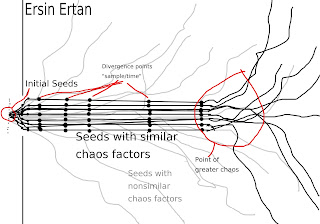### Chaos: A Very Short Introduction

Lenny Smith, Oxford University Press 2007
1. The emergence of chaos
2. Exponential growth, nonlinearity, common sense
3. Chaos in context: determinism, randomness and noise
4. Chaos in mathematical models
5. Fractals, strange attractors, and dimension(s)
6. Quantifying the dynamics of uncertainty
7. Real numbers, real observations, and computers
8. Sorry, wrong number: statistics and chaos
9. Predictability: does chaos constrain our forecasts?
10. Applied chaos: can we see through our models
11. Philosophy in chaos
Notable People: Isaac Newton, Pierre Laplace, Benjamin Franklin, Charles Darwin, meteorologist Ed Lorenz, ship captain Fitzroy, LeVerrier of France, Francis Galton, physicist James Clerk Maxwell, Alfred North Whitehead, Leonardo of Pisa,

Terms:
Chaos - behaviour of deterministic nonlinear, dynamical systems which are highly sensitive to initial conditions.(see Personal Notes 1)
Butterfly effect(sensitive dependence on initial conditions) - a phenomena in mathematics and science, systems where small differences now have huge consequences for the future. Behaviour that looks random but is not.
Sensitive dependence - the mathematical term for the butterfly effect.
Determinism - each decision, action, event is a result of the state before it.
Recurrence relation -  a sequence based on a rule that gives the next term as a function of the previous term(s)(determining a discrete dynamical system).
Nonlinearity - the input will show disproportionate output.
Burns effect - focus on our attempts to make rational decisions given only collections of different simulations under various imperfect models.
Exponential growth - a function where each successive step increases its value by a constant factor(multiplier.) The curve on a graph is a good model but not exact(gains precision with each succession, but never quite reaching its exact value)
Parameters - defines the constant of proportionality(between your multiplier and the unit.)
Time series - set of values reflecting change over time, most commonly in equal intervals.
Noise reduction - in perfect models, we can use future observations to learn about the past.
Recurrent trajectory - a sense of containment for chaos, where values are unconstrained but given enough time will return closer to the true state.(what does it mean by the true state? page 32)

Briefs:
Uncertainty is the active ingredient of forecasting. Forecasting lends itself to a visual, geometric understanding.
Chaos is the name of a mechanism allowing rapid growth of uncertainty in mathematical models. Chaos amplifies uncertainty and surprises forecasts, it is the description of the growth of uncertainty after the initial seed.
Noise gives rise to observational uncertainty, and chaos helps us understand how the small uncertainties can become large ones.
Forecasts of chaos using computer models may be subject to critical errors if the wrong data is provided or the system has a bug. It is important to have a fail safe or a quality control mechanism to cross reference results.
On average a small uncertainty will grow exponentially fast in a chaotic system.
Whatever the values of the parameters, at some point exponential growth will surpass linear growth.
Exponential growth is mathematically distinguished as the benchmark for defining chaos.
An error may grow exponentially, which has profound implications(expensive to verify) to the accuracy of the result. Error may be managed to 0% by knowing the initial conditions.
Using a infinitesimally small initial condition will allow uncertainty to stay small even within exponential growth.
Physical limits can constrain errors.

Personal Notes:
1. Is there something like the point of "greater chaos," assuming that within the timeline of a single initial seed, you define points of divergence(based on the comparisons with other initial seeds), give them weights, then aggregate and average them to find a chaos factor of the seed. Building on this way of measurement, if the distribution of chaos across multiple initial seeds is normalized(bell curve,) then we can conclude that the point of greater chaos is the midpoint or somewhere before, where the greatest amount of seeds yield the same or similar result(chaos factor), but then start to differ almost "exponential like" with respect to each other. Also note an interesting property of self-similarity with the period of initial seeding, and the point of greater chaos(is there a relation?), it's a matter of resolution, since both can be considered points of greater chaos, and if you zoom out, then the "POGC" may even be further away, assuming the number of seeds(seed resolution) has increased as well.Problems I have with my own diagram: at every point(not divergence point) there should be an infinite amount of lines moving away from the our chaos factor range, thus how we picked the point of greater chaos was rather arbitrary. At what resolution or sample rate for the divergence points is also a hard to define, initialize, generalize, I would assume it would be specific to the problem. Will determinism, solve these issues? Is the real chaos just the point of greater chaos?
2. Test
Chapter 3 chaos in context determinism, randomness, and noise